## EMF and Torque:

EMF and Torque – It was shown in Sec. 5.2 (Fig. 5.14 (a)) that in a dc machine the magnetic structure is such that the flux density wave in the air-gap is flat-topped with quarter-wave symmetry so long as the armature is not carrying any current. It will be seen in Sec. 7.6 that the flux density wave gets distorted when the armature carries current (armature reaction effect destroys quarter-wave symmetry). However, this fact does not affect the constancy of emf (between brushes) and torque developed by the machine with magnitudes of each of these being determined by the flux/pole independent of the shape of the B-wave.This number is fixed independent of the armature rotation; as one coil moves out of the parallel path another comes in and takes its place (see Sec. 6.3). Thus, the parallel path emf which equals the armature/ emf is given by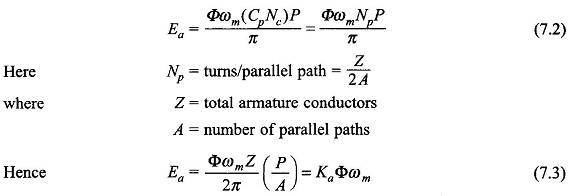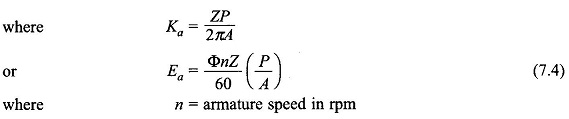Ea remains constant (dc) by virtue of fixed coils per parallel path independent of rotation of armature (commutation action). It is also observed that Ea depends upon the flux/pole and not upon the shape of the flux density wave.

## Torque Equation

Figure 7.3 shows the flux density wave in the air-gap and the conductor current
distribution in the developed armature for one pole-pair. It is immediately seen that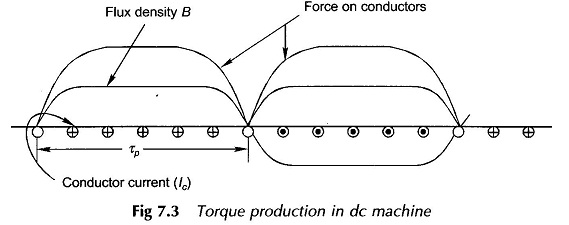the force on conductors is unidirectional. Each conductor as it moves around with the armature experiences a force whose time variation is a replica of the B-wave. Therefore, the average conductor force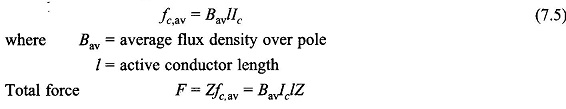This force (and therefore torque) is constant (independent of time) because both the flux density wave and current distribution are fixed in space at all times. Now the torque developed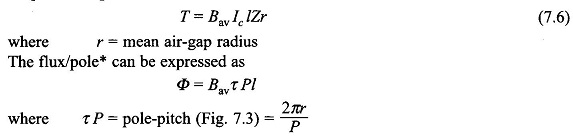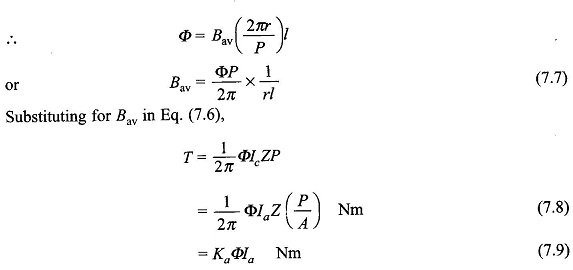It is, therefore, seen that the machine torque is uniform for given flux/pole and armature current. Further, it is independent of the shape of the B-wave, which in fact gets distorted by the armature mmf when it carries current (see Sec. 7.5).

It is convenient to use force on each conductor in deriving the expression for armature torque. However, the mechanism of torque production is different in an actual machine in which conductors are placed in armature slots. The force is produced by the interaction of the main flux and the flux produced by current carrying conductors placed in armature slots. Due to the large reluctance of the air-path of slots, the main flux passing through the conductors is negligible and so is the force acting on the conductor. Force is produced mainly by the distortion of the flux lines passing through the teeth, and this force acts on the teeth of the armature as shown in Fig. 7.4. It is rather fortunate that there is very little force acting on conductors. If all the force were to act on conductors, it would crush the insulation between conductors and slots.## Power Balance

Mechanical power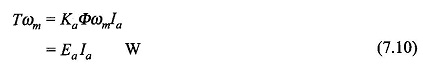This is nothing but a statement of energy conservation, i.e. electrical and mechanical powers must balance in a machine.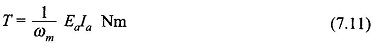## Linear Magnetization

If the magnetic circuit of the machine is assumed linear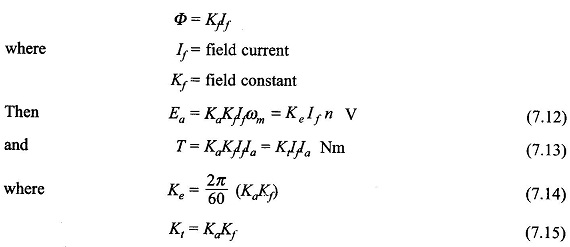The derivation of torque developed (Eq. (7.8)) using magnetic field interaction is carried out in Sec. 7.5 after armature reaction ampere turns are determined